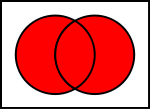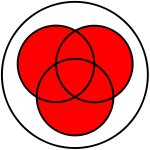# Union (set theory)

Union (set theory)Union of two sets:$~A \cup B$Union of three sets:$~A \cup B \cup C$

In set theory, the union (denoted as ∪) of a collection of sets is the set of all distinct elements in the collection. The union of a collection of sets$S_1, S_2, S_3, \dots , S_n\,\!$ gives a set$S_1 \cup S_2 \cup S_3 \cup \dots \cup S_n$.

## Definition

The union of two sets A and B is the collection of points which are in A or in B (or in both):$A \cup B = \{ x: x \in A \,\,\,\textrm{ or }\,\,\, x \in B\}$

A simple example:

A = {1,2,3,4}
B = {5,6,7,8}$A \cup B = \{1,2,3,4,5,6,7,8\}$

Other more complex operations can be done including the union, if the set is for example defined by a property rather than a finite or assumed infinite enumeration of elements. As an example, a set could be defined by a property or algebraic equation, which is referred to as a solution set when resolved. An example of a property used in a union would be the following:

A = {x is an even number, x > 1}
B = {x is an odd number, x > 1}$A \cup B = \{2,3,4,5,6,...\}$

If we are then to refer to a single element by the variable "x", then we can say that x is a member of the union if it is an element present in set A or in set B, or both.

Sets cannot have duplicate elements, so the union of the sets {1, 2, 3} and {2, 3, 4} is {1, 2, 3, 4}. Multiple occurrences of identical elements have no effect on the cardinality of a set or its contents. The number 9 is not contained in the union of the set of prime numbers {2, 3, 5, 7, 11, …} and the set of even numbers {2, 4, 6, 8, 10, …}, because 9 is neither prime nor even.

### Algebraic properties

Binary union is an associative operation; that is,

A ∪ (BC) = (AB) ∪ C.

The operations can be performed in any order, and the parentheses may be omitted without ambiguity (i.e. either of the above can be expressed equivalently as ABC). Similarly, union is commutative, so the sets can be written in any order. The empty set is an identity element for the operation of union. That is, A ∪ {} = A, for any set A. In terms of the definitions, these facts follow from analogous facts about logical disjunction.

Together with intersection and complement, union makes any power set into a Boolean algebra. For example, union and intersection distribute over each other, and all three operations are combined in De Morgan's laws. Replacing union with symmetric difference gives a Boolean ring instead of a Boolean algebra.

## Forms

### Finite unions

More generally, one can take the union of several sets at once. The union of A, B, and C, for example, contains all elements of A, all elements of B, and all elements of C, and nothing else. Formally, x is an element of ABC if and only if x is in A, B, or C.

Union is an associative operation, it doesn't matter in what order unions are taken. In mathematics a finite union means any union carried out on a finite number of sets: it doesn't imply that the union set is a finite set.

### Arbitrary unions

The most general notion is the union of an arbitrary collection of sets. If M is a set whose elements are themselves sets, then x is an element of the union of M if and only if for at least one element A of M, x is an element of A. In symbols:$x \in \bigcup\mathbf{M} \iff \exists A \in \mathbf{M},\ x \in A.$

That this union of M is a set no matter how large a set M itself might be, is the content of the axiom of union in axiomatic set theory.

This idea subsumes the above paragraphs, in that for example, ABC is the union of the collection {A,B,C}. Also, if M is the empty collection, then the union of M is the empty set. The analogy between finite unions and logical disjunction extends to one between arbitrary unions and existential quantification.

The notation for the general concept can vary considerably, such as the following:$\bigcup \mathbf{M},$$\bigcup_{A\in\mathbf{M}} A.$$\bigcup_{i\in I} A_{i},$

which refers to the union of the collection {Ai : i is in I}. Here I is an index set, and Ai is a set for every i in I. In the case that the index set I is the set of natural numbers, the notation is analogous to that of infinite series:$\bigcup_{i=1}^{\infty} A_{i}.$

When formatting is difficult, this can also be written "A1A2A3 ∪ ···". (This last example, a union of countably many sets, is very common in analysis; for an example see the article on σ-algebras.) Whenever the symbol "∪" is placed before other symbols instead of between them, it is of a larger size.

Intersection distributes over infinitary union, in the sense that$A \cap \bigcup_{i\in I} B_{i} = \bigcup_{i\in I} (A \cap B_{i}).$

Infinitary union can be combined with infinitary intersection to get the law$\bigcup_{i\in I} \bigg(\bigcap_{j\in J} A_{i,j}\bigg) \subseteq \bigcap_{j\in J} \bigg(\bigcup_{i\in I} A_{i,j}\bigg)$.

Wikimedia Foundation. 2010.

### Look at other dictionaries:

• Set theory of the real line — is an area of mathematics concerned with the application of set theory to aspects of the real numbers. For example, one knows that all countable sets of reals are null, i.e. have Lebesgue measure 0; one might therefore ask the least possible size …   Wikipedia

• set theory — the branch of mathematics that deals with relations between sets. [1940 45] * * * Branch of mathematics that deals with the properties of sets. It is most valuable as applied to other areas of mathematics, which borrow from and adapt its… …   Universalium

• Set theory — This article is about the branch of mathematics. For musical set theory, see Set theory (music). A Venn diagram illustrating the intersection of two sets. Set theory is the branch of mathematics that studies sets, which are collections of objects …   Wikipedia

• Set theory (music) — Example of Z relation on two pitch sets analyzable as or derivable from Z17 (Schuijer 2008, p.99), with intervals between pitch classes labeled for ease of comparison between the two sets and their common interval vector, 212320. Musical set… …   Wikipedia

• Zermelo set theory — Zermelo set theory, as set out in an important paper in 1908 by Ernst Zermelo, is the ancestor of modern set theory. It bears certain differences from its descendants, which are not always understood, and are frequently misquoted. This article… …   Wikipedia

• Naive set theory — This article is about the mathematical topic. For the book of the same name, see Naive Set Theory (book). Naive set theory is one of several theories of sets used in the discussion of the foundations of mathematics. The informal content of… …   Wikipedia

• Constructive set theory — is an approach to mathematical constructivism following the program of axiomatic set theory. That is, it uses the usual first order language of classical set theory, and although of course the logic is constructive, there is no explicit use of… …   Wikipedia

• Descriptive set theory — In mathematical logic, descriptive set theory is the study of certain classes of well behaved subsets of the real line and other Polish spaces. As well as being one of the primary areas of research in set theory, it has applications to other… …   Wikipedia

• Quasi-set theory — is a formal mathematical theory of collections of indistinguishable objects, mainly motivated by the assumption that certain objects treated in quantum physics are indistinguishable. Quasi set theory is closely related to, yet distinct from,… …   Wikipedia

• General set theory — (GST) is George Boolos s (1998) name for a three axiom fragment of the canonical axiomatic set theory Z. GST is sufficient for all mathematics not requiring infinite sets, and is the weakest known set theory whose theorems include the Peano… …   Wikipedia